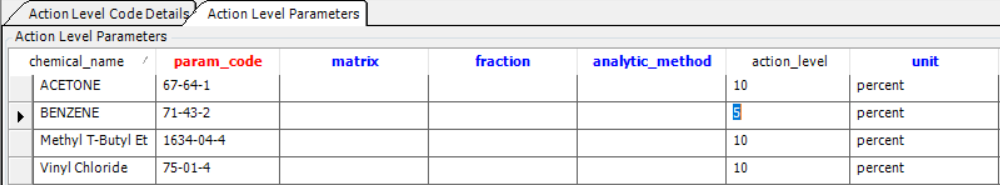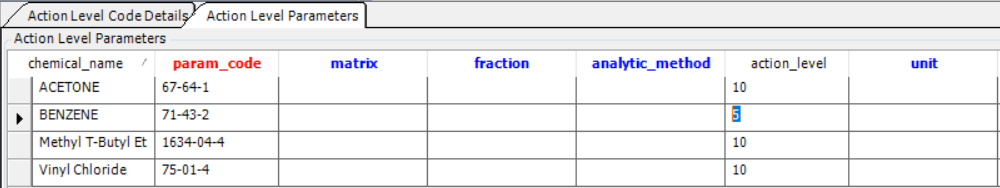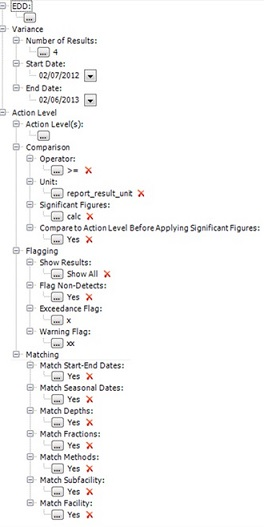﻿ Action Level Exceedance II - Percent Variance

# Action Level Exceedance II - Percent Variance

Report Name: Action Level Exceedance II - Percent Variance*

*previously called (Beta) Action Level Exceedance - Percent Variance

Report Type: Standard Report

Description: The Action Level Exceedance II - Percent Variance Report is designed to flag analytical results within a given EDD that vary by more than the listed percentage from the historical average for each chemical and location.

The allowable percentage of variation is listed for each chemical in DT_ACTION_LEVEL_PARAMETER. An action_level and action_level_min are calculated by adding and subtracting the percentage amount from the average of the historical result values for each location.

In addition, the report can be run as an EQuIS Information Agent (EIA) as each new EDD is loaded (see EZView for information on setting a report up as an EIA).

Report Parameters

Variance Parameters:The historical result average is determined from the Variance input parameters:

Number of Results: Count of results to include in the historical average calculation, starting from the most recent within the specified date range.

Start/End Dates: The sample date range of results used to determine the historical result average.

Action Level Parameters:Action Level(s): An action level must be selected for the report to run. For this report, only one action level may be selected. The other Action Level parameters work as in the other Action Level Exceedance II reports.

Example Percent Variance Action Level:Example Report Output:Notes: •A summary of calculations are recorded in alp_remark consisting of  Variance, Average of Values and Number of Values.•If the number of historical results in the date range specified is less than the entered number for an EDD result, the alp_remark of the output will show how many historical results were used in the calculations. •If the number of historical results in the date range specified is more than the entered number for an EDD result, the most recent historical results are used in the calculations.•The data of the selected EDD itself will never be used in the historical average calculation, even if the sample dates of the EDD are within the Variance date range.•The SYS_LOC_CODE, CAS_RN and MATRIX_CODE of the EDD results are used to find matching historical results.

The report action_level and action_level_min are calculated as follows:

action_level = result average + (percent * result average)

action_level_min = result average - (percent * result average)

In the above example, the calculations would look like this:

B-30:

oaction_level = 15.67 + (0.05 * 15.67) = 16.5

oaction_level_min = 15.67 - (0.05 * 15.67) = 14.9

B-31:

oaction_level = 15.31 + (0.05 * 15.31) = 16.1

oaction_level_min = 15.31 - (0.05 * 15.31) = 14.5

B-32:

oaction_level = 0.0 + (0.05 * 0.0) = 0.0

oaction_level_min = 0.0 - (0.05 * 0.0) = 0.0

Variation:

Example Action Level without "percent" or "%" in the DT_ACTION_LEVEL_PARAMETER.UNIT:Returns the following report output from the same data:In the above example, the calculations would look like this:

B-30:

oaction_level = 15.67 + 5 = 20.7

oaction_level_min = 15.67 - 5 = 10.7

B-31:

oaction_level = 15.31 + 5 = 20.3

oaction_level_min = 15.31 - 5 = 10.3

B-32:

oaction_level = 0.0 + 5 = 5.0

oaction_level_min = 0.0 - 5 = -5.0

Report Dependencies: This report depends on the Analytical Results II Report and must have the 50943.dll available to work properly.

File Name: EarthSoft.Reports.Library.51729.dll

Contact support@earthsoft.com for a direct file link.

Language: English

Input Parameters: A selection made to customize the output of a report.

Input Parameters

Input Parameter Screenshot

Output Columns

Analytical Results

Variance

Number of Results

Start Date

End Date

Action Level

Comparison

Operator

Unit

Significant Figures

Compare to Action Level Before Analyzing Significant Figures

Flagging

Show Results

Flag Non-detects

Exceedance Flag

Warning Flag

Matching

Match Start - End Dates

Match Seasonal Dates

Match Depths

Match Fractions

Match Methods

Match Subfacility

Match FacilityContact

Note: *refers to hidden parameters

Tables: DT_ACTION_LEVEL, DT_ACTION_LEVEL_PARAMETER, DT_LOCATION, DT_SAMPLE, DT_SUBFACILITY, DT_TEST, RT_GROUP, ST_REPORT, ST_USER_REPORT, DT_SAMPLE, DT_TEST, ST_EDD_BATCH, ST_REPORT, ST_USER_REPORT, DT_RESULT, DT_SAMPLE, DT_TEST, ST_EDD_BATCH, FACILITY_ID, FACILITY_CODE, SYS_LOC_CODE, LOC_NAME, SAMPLE_ID, SYS_SAMPLE_CODE, SAMPLE_NAME, SAMPLE_DATE, SAMPLE_TYPE_CODE, START_DEPTH, END_DEPTH, DEPTH_UNIT, MATRIX_CODE, TASK_CODE, PARENT_SAMPLE_CODE, ANALYSIS_LOCATION, LAB_SAMPLE_ID, LAB_MATRIX_CODE, LAB_NAME_CODE, ANALYTIC_METHOD, ANALYSIS_DATE, COLUMN_NUMBER, FRACTION, TEST_TYPE, PREP_METHOD, LEACHATE_METHOD, LEACHATE_DATE, LAB_SDG, PERCENT_MOISTURE, DILUTION_FACTOR, TEST_ID, CAS_RN, CHEMICAL_NAME, ORGANIC_YN, REPORT_RESULT_TEXT, REPORT_RESULT_VALUE, REPORT_RESULT_UNIT, REPORT_RESULT_LIMIT, REPORTABLE_RESULT, DETECT_FLAG, INTERPRETED_QUALIFIERS,  VALIDATOR_QUALIFIERS, LAB_QUALIFIERS, QUANTITATION_LIMIT, METHOD_DETECTION_LIMIT, REPORTING_DETECTION_LIMIT, DETECTION_LIMIT_UNIT, APPROVAL_CODE, RESULT_TEXT, RESULT_NUMERIC, RESULT_UNIT, RESULT_TYPE_CODE, X_COORD, Y_COORD, Z_COORD_AVG, ZFROM, ZTO, LONGTITUDE, LATITUDE, LOC_GROUP_CODE, LOC_REPORT_ORDER

Database Type: SQL

Database Schema: Standard (no custom or add-on schemas required)

Database Version: EQuIS 5.6+

Data Requirements: N/A

Report Requirements: Excel

Revision History: In EQuIS 7 the revisions explained below have been completed.

1.If there are samples without sample_date, their results may be used to calculate action_level. For example, if Number of Results of Variance is selected as four, and there are three historic samples within the selected date range and one sample of no sample date, the results of the four samples are used to calculate action_level. Another issue is that if samples do not have matrix_code, the report does not calculate action_level from Variance. These issues are fixed (Case 138773).

2.The report is modified so that it can be triggered by EIA. The report generates a result based on the ebatch created by the EDD for a saved user report whose EIA is turned on (Case 135710).

Output Type: Excel